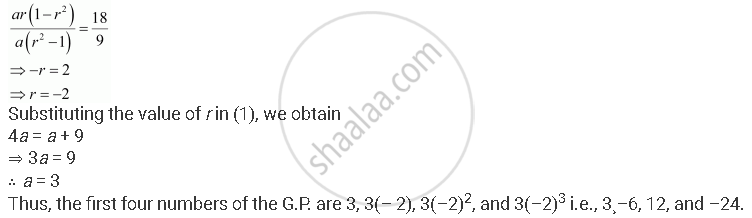CBSE (Arts) Class 11CBSE
Share
Notifications

View all notifications
Books Shortlist
Your shortlist is empty

# Find Four Numbers Forming a Geometric Progression in Which Third Term is Greater than the First Term by 9, and the Second Term is Greater than the 4th by 18. - CBSE (Arts) Class 11 - Mathematics

Login
Create free account

Forgot password?
ConceptGeometric Progression (G. P.)

#### Question

Find four numbers forming a geometric progression in which third term is greater than the first term by 9, and the second term is greater than the 4th by 18.

#### Solution

Let a be the first term and r be the common ratio of the G.P.

a1 = aa2 = ara3 = ar2a4 = ar3

By the given condition,

a3 = a1 + 9

⇒ ar2 = a + 9 … (1)

a2 = a4 + 18

⇒ ar ar3 + 18 … (2)

From (1) and (2), we obtain

a(r2 ­­– 1) = 9 … (3)

ar (1– r2) = 18 … (4)

Dividing (4) by (3), we obtainIs there an error in this question or solution?

#### APPEARS IN

NCERT Solution for Mathematics Textbook for Class 11 (2018 to Current)
Chapter 9: Sequences and Series
Q: 21 | Page no. 193
Solution Find Four Numbers Forming a Geometric Progression in Which Third Term is Greater than the First Term by 9, and the Second Term is Greater than the 4th by 18. Concept: Geometric Progression (G. P.).
S